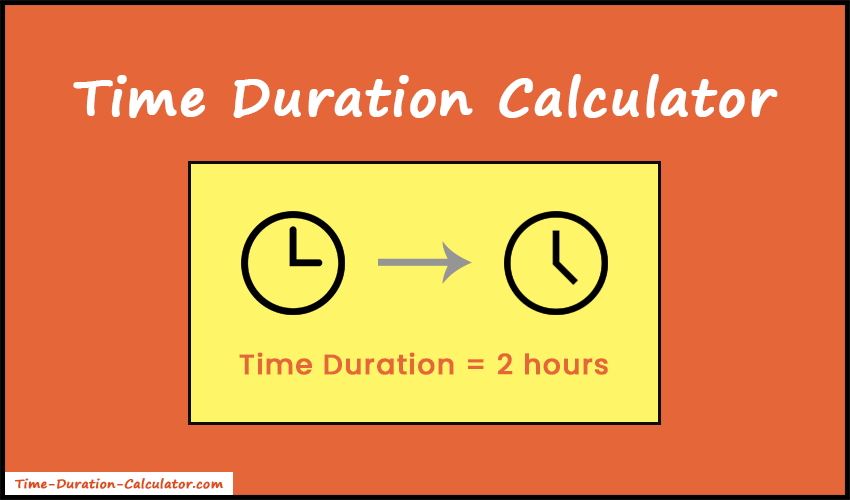# Time Duration Calculator

The time duration calculator lets you find the duration between two times in hours, minutes, and seconds.

##### More Useful Tools
Date Duration Calculator Birthday Calculator

Time Duration Calculator is a free and accurate online tool to calculate the duration between two times. Mostly, it is used by payroll managers, employees, and supervisors to calculate how long they worked on a project or how much time does the task last. In short, it's a time-tracking tool that lets you know how much time you have left. In addition, you can use it to compute the daily work hours and also use the timesheet calculator to create a full timecard.

Just enter the start time and end time in the input boxes and choose the appropriate AM or PM option. That's all. Our system will start calculating and give you the final result in hours, minutes, and seconds. Also, the result will be 100% accurate.

Moreover, you can calculate the days between two dates using the day calculator.## How Does the Time Duration Calculator Works?

• Time duration calculator takes two input values from the users. That's the Start time and the End time. Most importantly, the input values should be in a 12-hour format such as "HH:MM (AM/PM)".
• After entering all the values, just press the "Calculate" button.
• After that, our calculator will subtract the start time from the end time to get the duration between two given times.
• Finally, the tool breaks down the duration result into different time units and shows results in hours and minutes, total minutes, and total seconds.

### How to Calculate Time Duration?

Calculating the duration between two times is complex. Because it depends on the hours and minutes of the two given times being compared.

Also, we need to convert the hours into the 24-hour time format to calculate the duration. AM hours will remain the same for both 12-hour and 24-hour formats. But for PM hours, we need to add 12 to the number of hours to get the 24-hour time. For example, 04:25 PM will be 16:25 in 24-hour format.

So, let's take some examples to understand how to determine the number of hours and minutes between two times. Also, we will take the start time and end time within the same day.

#### Example 1:

Find the duration between the following times:
Start time = 09:35 AM
End time = 01:45 PM

##### Solution:

Firstly, we will convert both times into a 24-hour format.

So, the Start time would be 09:35, and the End time would be 13:45.

Now subtract the start time hours from the end time hours.

13 - 9 = 4 hours.

Similarly, subtract the start time minutes from the end time minutes.

45 - 35 = 10 minutes.

Finally, we get the time duration result: 4 hours, 10 minutes.

#### Example 2:

Suppose, Start time = 03:45 PM and End time = 06:25 PM. Calculate the time duration between given times.

##### Solution:

Here, both time is in PM. So, let's convert it into 24-hour time.

After converting,

Start time = 15:45
End time = 18:25

Here, end time minutes are less than start time minutes. So, we will subtract 1 hour from the end time hours and add the 60 minutes to the end time minutes.

18 - 1 = 17
25 + 60 = 85

As a result, we get End time = 17:85

Now subtract the start time hours from the end time hours.

17 - 15 = 2 hours.

Similarly, subtract the start time minutes from the end time minutes.

85 - 45 = 40 minutes.

Finally, we get the duration result: 2 hours, 40 minutes.

As you can see, the manual calculation is complex and time taking. Therefore, it's better to use our time duration calculator to make this task easy, fast, and accurate.